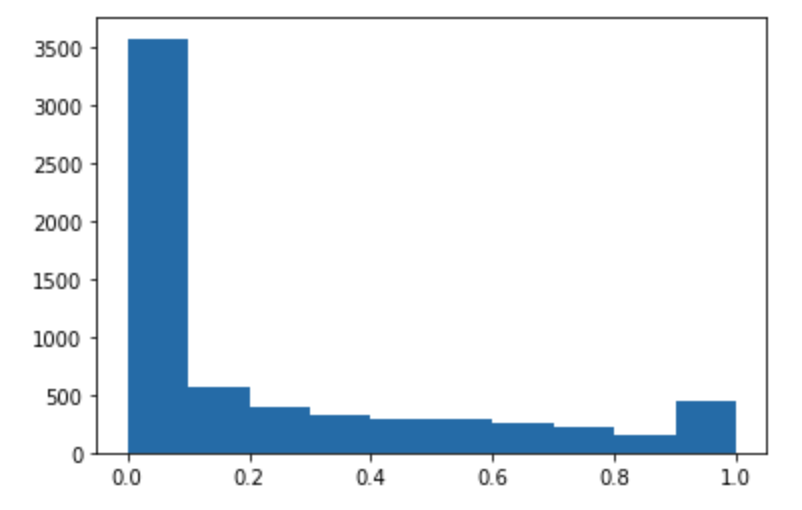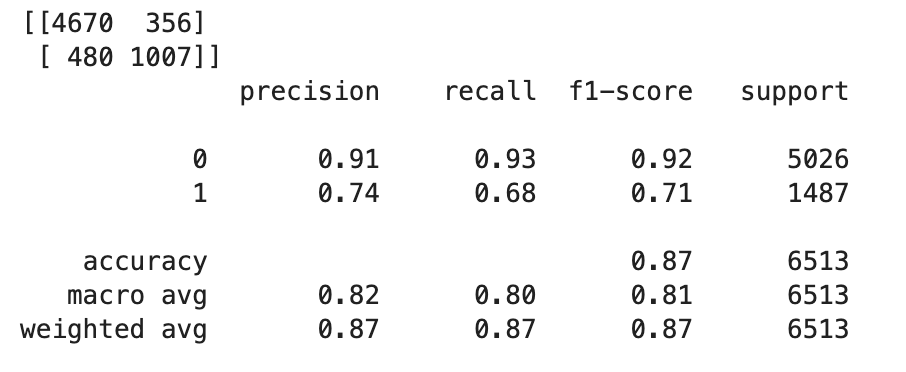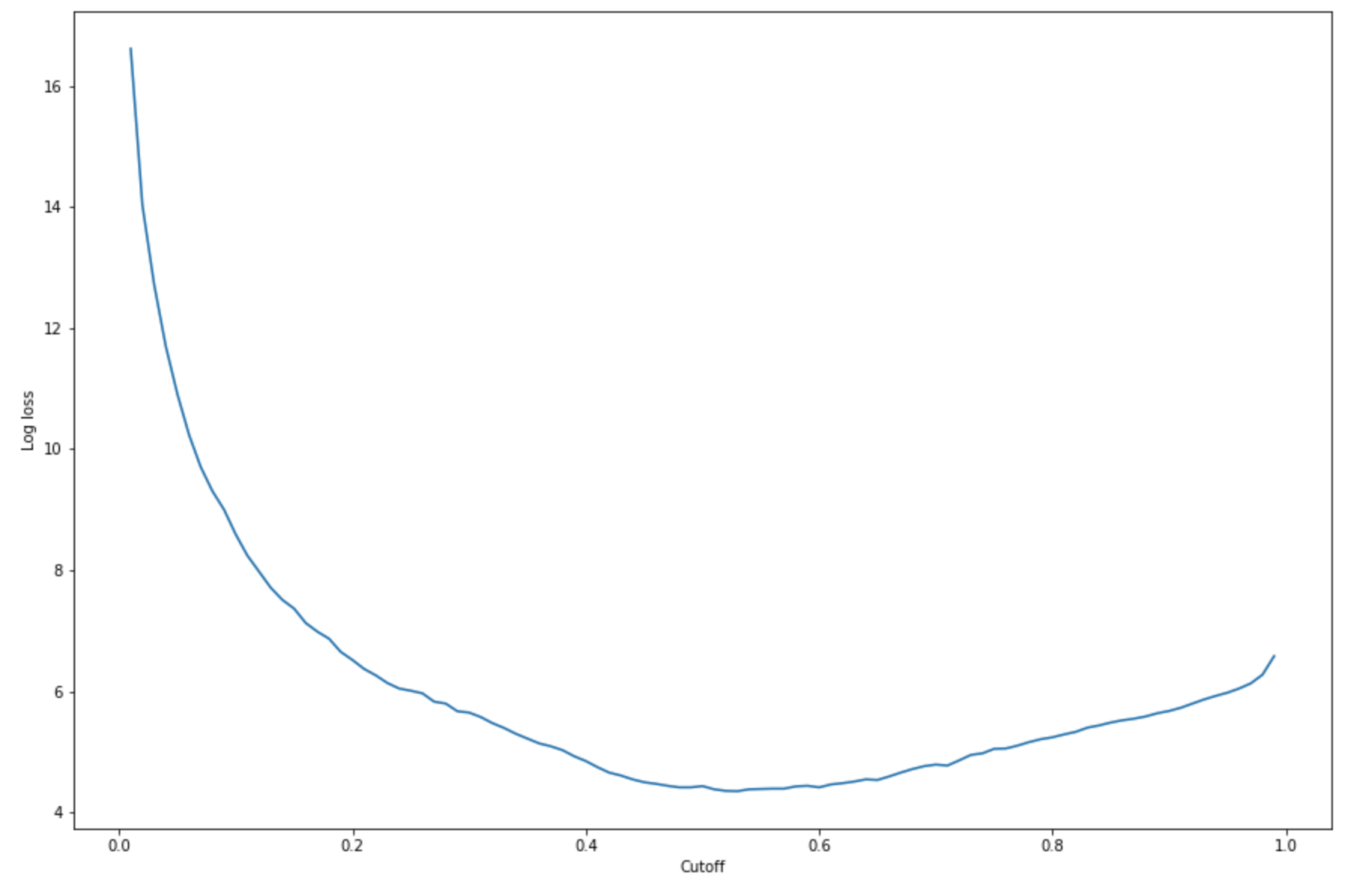Amazon Web Services 文档中描述的 Amazon Web Services 服务或功能可能因区域而异。要查看适用于中国区域的差异，请参阅中国的 Amazon Web Services 服务入门

# 第 6 步：评估模型

## 评估已部署到的模型 SageMaker 托管服务

1. 设置以下函数来预测测试集中的每一行。在以下示例代码中，`rows`参数是指定一次要预测的行数。您可以更改它的值，以执行充分利用实例硬件资源的批量推断。

``````import numpy as np
def predict(data, rows=1000):
split_array = np.array_split(data, int(data.shape / float(rows) + 1))
predictions = ''
for array in split_array:
predictions = ','.join([predictions, xgb_predictor.predict(array).decode('utf-8')])
return np.fromstring(predictions[1:], sep=',')``````
2. 运行以下代码来预测测试数据集并绘制直方图。您只需要测试数据集的要素列，不包括实际值的第 0 列。

``````import matplotlib.pyplot as plt

predictions=predict(test.to_numpy()[:,1:])
plt.hist(predictions)
plt.show()``````3. 预测值是浮点型。确定`True`要么`False`根据浮点值，你需要设置截止值。如以下示例代码所示，使用 Scikit-Learn 库返回输出混淆指标和截止值为 0.5 的分类报告。

``````import sklearn

cutoff=0.5
print(sklearn.metrics.confusion_matrix(test.iloc[:, 0], np.where(predictions > cutoff, 1, 0)))
print(sklearn.metrics.classification_report(test.iloc[:, 0], np.where(predictions > cutoff, 1, 0)))``````

这应返回以下混淆矩阵：4. 要找到与给定测试集合的最佳截止值，请计算 Logistic 回归的对数损失函数。对数丢失函数被定义为逻辑模型的负对数可能性，该模型返回其地面真相标签的预测概率。下面的示例代码以数字方式和迭代方式计算对数损失值 (`-(y*log(p)+(1-y)log(1-p)`)，其中`y`是真正的标签`p`是对应测试样本的概率估计。它返回了日志丢失与截止图。

``````import matplotlib.pyplot as plt

cutoffs = np.arange(0.01, 1, 0.01)
log_loss = []
for c in cutoffs:
log_loss.append(
sklearn.metrics.log_loss(test.iloc[:, 0], np.where(predictions > c, 1, 0))
)

plt.figure(figsize=(15,10))
plt.plot(cutoffs, log_loss)
plt.xlabel("Cutoff")
plt.ylabel("Log loss")
plt.show()``````

这应返回以下日志损失曲线。5. 使用 NumPy 查找错误曲线的最小点`argmin``min`函数：

``````print(
'Log loss is minimized at a cutoff of ', cutoffs[np.argmin(log_loss)],
', and the log loss value at the minimum is ', np.min(log_loss)
)``````

应返回：```Log loss is minimized at a cutoff of 0.53, and the log loss value at the minimum is 4.348539186773897```.

您可以估计成本函数作为替代方案，而不是计算和最大限度地减少日志丢失函数。例如，如果您想训练模型来对业务问题（例如客户流失预测问题）执行二进制分类，则可以为混淆矩阵的元素设置权重并相应地计算成本函数。